# Month: February 2019

Each journal article in MathSciNet is tagged with one or more MSC classification numbers. Here are the graphics for few of them around analysis, probability, and statistics (this is not exclusive since an article can contain multiple numbers).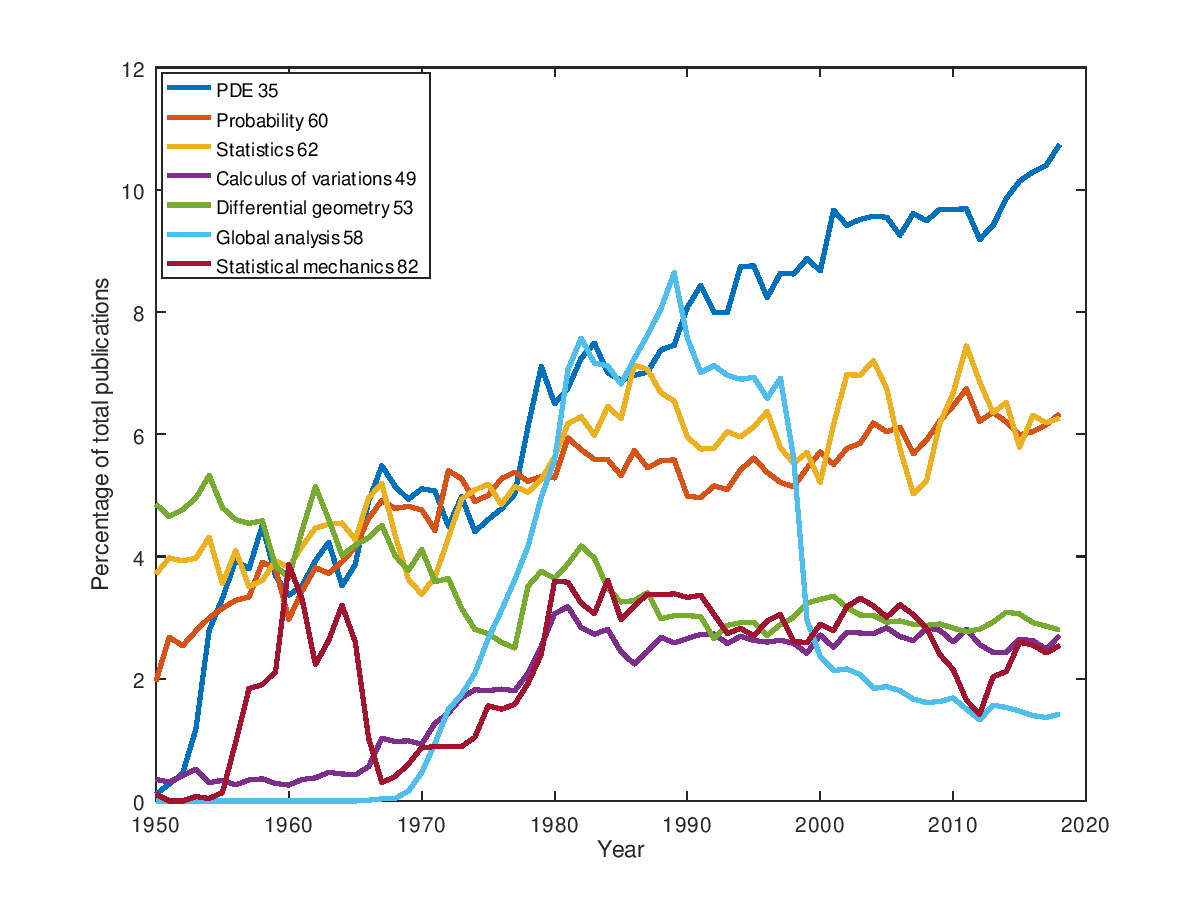Same kind of graphics with more data (beware that the colors are not the same):I have learned recently from my colleague Patrick Cattiaux via Michel Ledoux that is it very easy to get from MathSciNet (MSN) the total number of publications per year. Actually I already knew that from my colleague Jean Dolbeault. It is tempting to play with this data. Here are the graphics obtained with few lines of scripting, for journal articles, books, and proceedings. The numbers depend on the selection of titles made by the Mathematical Reviews, which may vary in time, and which can be seen as a good definition of mathematics at large.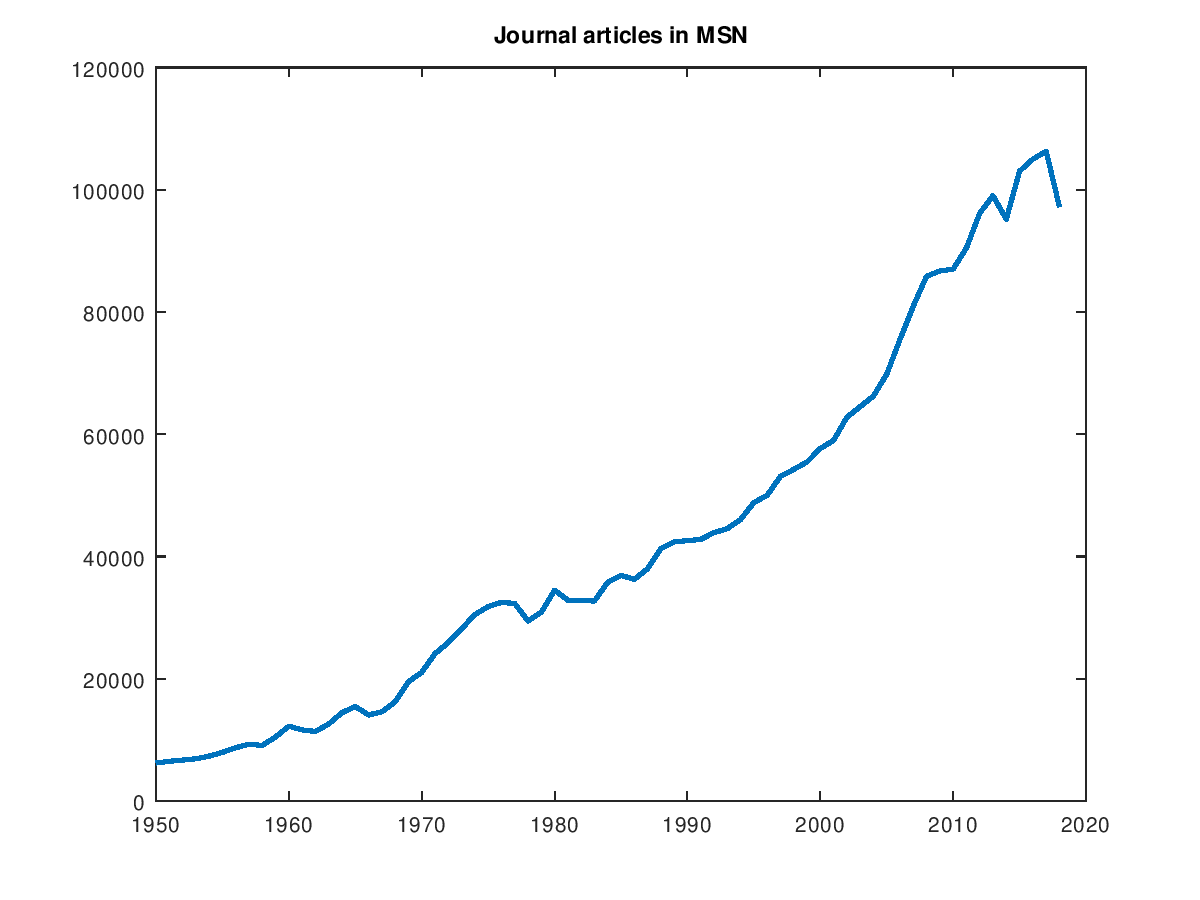Now the same with the Zentralblatt MATH  (ZBM) database: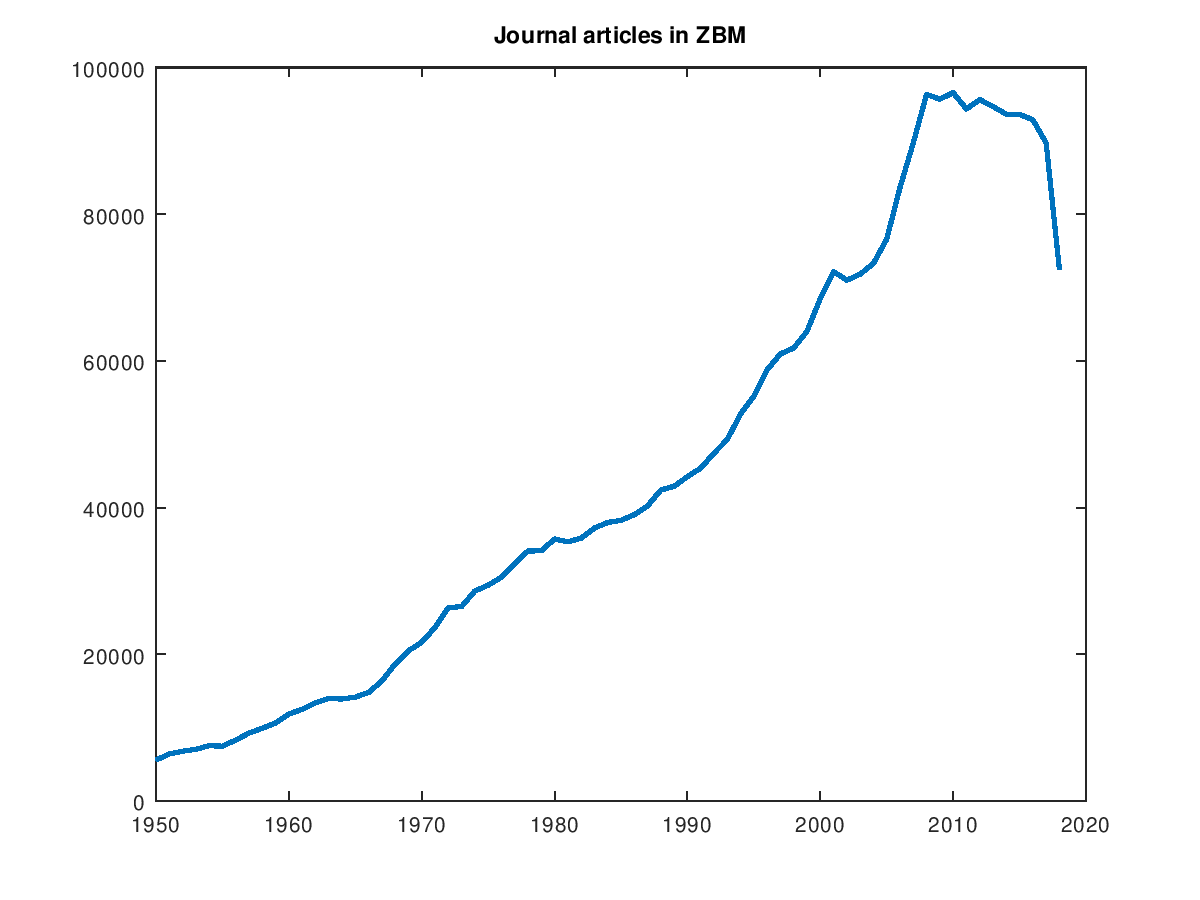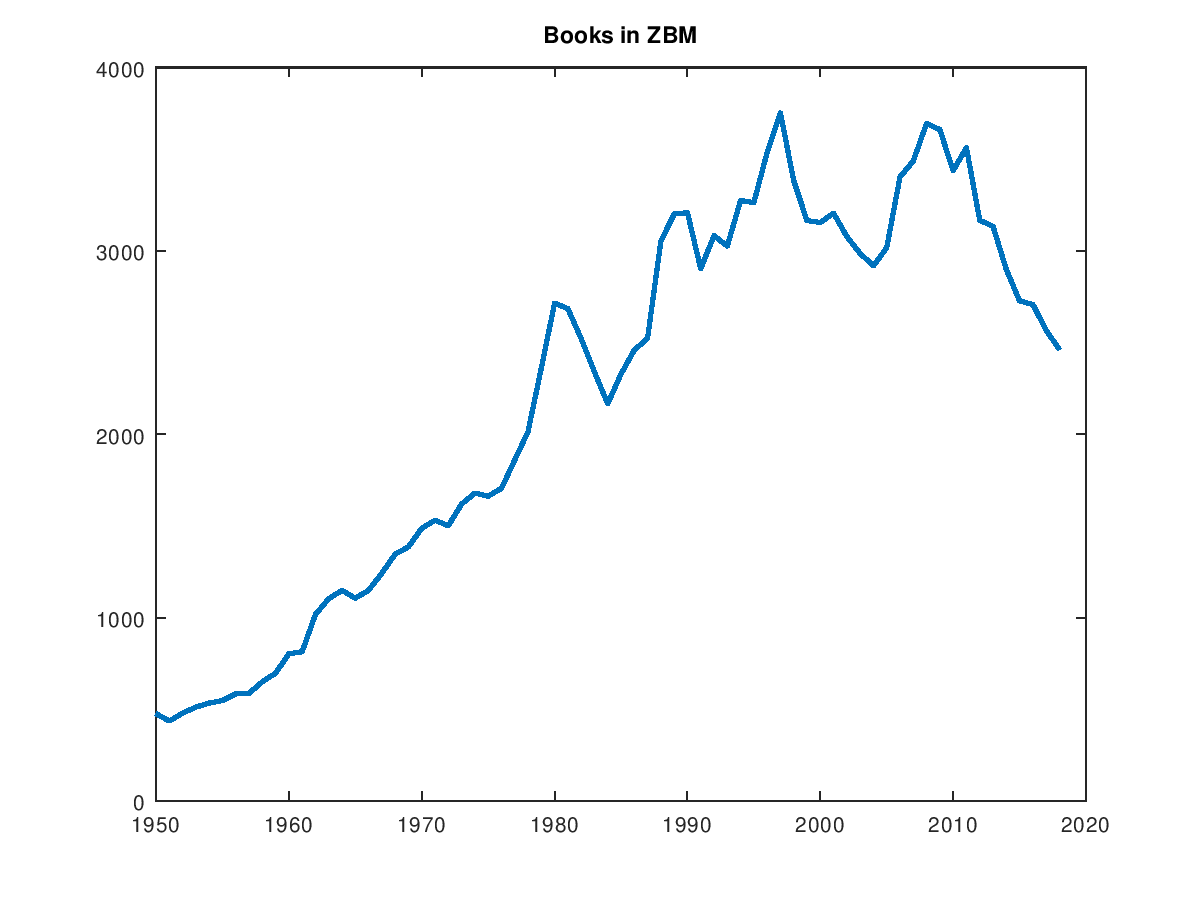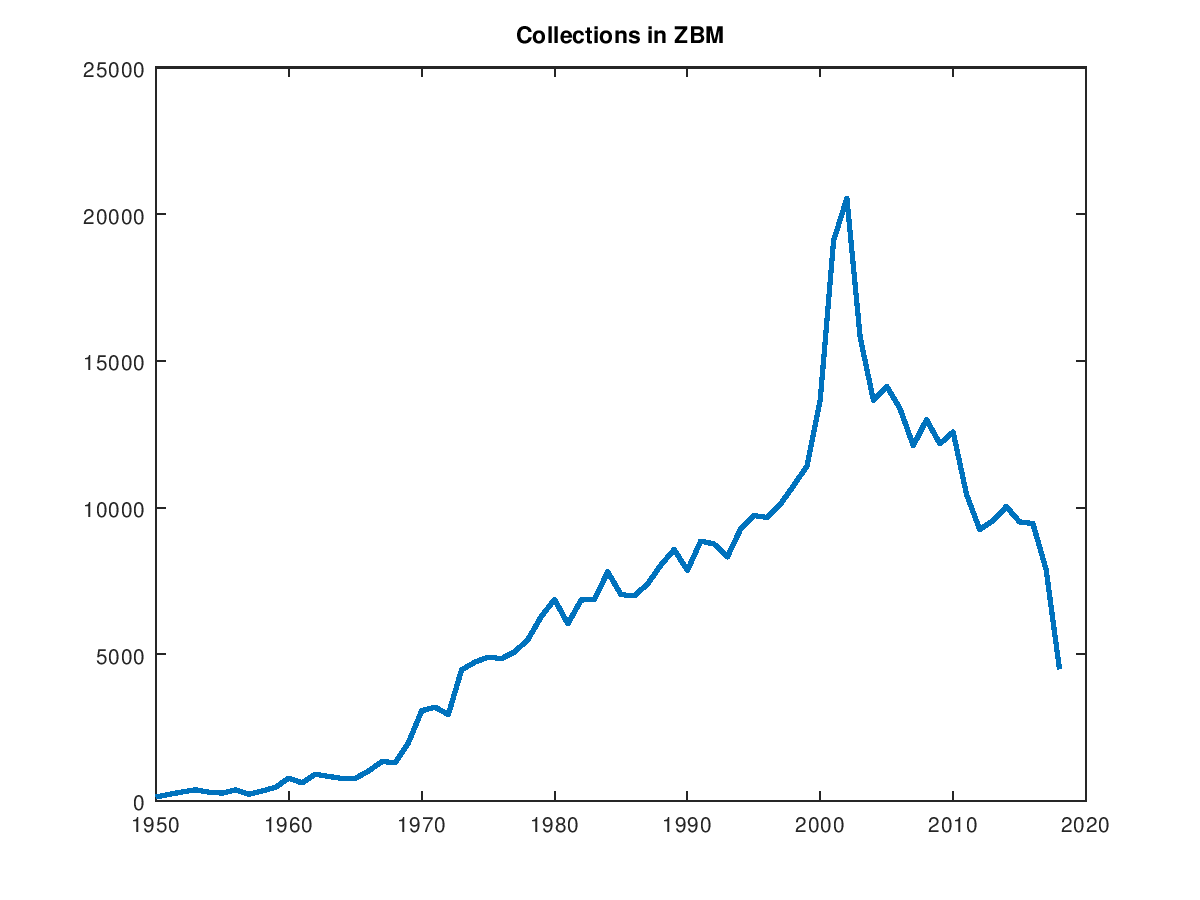This post is about a remarkable projective property of certain Boltzmann-Gibbs measures.

The model. Let $d\geq1$ and $g:\mathbb{R}^d\to(-\infty,+\infty]$ be continuous with $g(x)<\infty$ for all $x\neq0$. For all $\beta>0$, $n\geq2$, let $P_n$ be the probability measure on $(\mathbb{R}^d)^n$ with density proportional to $$(x_1,\ldots,x_n)\in(\mathbb{R}^d)^n\mapsto\exp(-\beta H(x_1,\ldots,x_n))$$ where $$H(x_1,\ldots,x_n)=\sum_{i=1}^n\frac{1}{2}|x_i|^2+\sum_{i\neq j}g(x_i-x_j).$$ Let $$X=(X_{n,1},\ldots,X_{n,n})\sim P_n.$$

A projection. Let $p:\mathbb{R}^d\to\mathbb{R}^d$ be an orthogonal projection on a subspace $E\subset\mathbb{R}^d$. Let $\pi$ and $\pi^\perp$ be the orthogonal projections on the subspaces $$L=\{(p(z),\ldots,p(z))\in(\mathbb{R}^d)^n:z\in\mathbb{R}^d\}\quad\text{and}\quad L^\perp.$$ We have, for all $x\in(\mathbb{R}^d)^n$,

$$\pi(x)=(p(s(x)),\ldots,p(s(x)))\quad\text{where}\quad s(x):=\frac{x_1+\cdots+x_n}{n}\in\mathbb{R}^d.$$

Indeed, we have
$$L^\perp=\{(x_1,\ldots,x_n)\in(\mathbb{R}^d)^n:(x_1+\cdots+x_n)\cdot p(z)=0\text{ for all }z\in\mathbb{R}^d\},$$
and for all $x=(x_1,\ldots,x_n)\in(\mathbb{R}^d)^n$ and all $(z,\ldots,z)\in L$, $z\in E$, we have
\begin{align}((x_1,\ldots,x_n)-(p(s(x)),\ldots,p(s(x))))\cdot(z,\ldots,z) &=\sum_{i=1}^nx_i\cdot z-np(s(x))\cdot z\\&=\sum_{i=1}^nx_i\cdot z-\sum_{i=1}^np(x_i)\cdot z\\&=\sum_{i=1}^n(x_i-p(x_i))\cdot z\\&=\sum_{i=1}^n0=0.\end{align}

Example 1: if $E=\mathbb{R}^d$ then $p(x)=x$.

Example 2 : $E=\mathbb{R}z$ for $z\in\mathbb{R}^d$ with $|z|=1$. Then $p(x)=(x\cdot z)z$.​​

The statement.  If all the ingredients are as above, then:

• $\pi(X)$ and $\pi^\perp(X)$ are independent random vectors;
• $\pi(X)$ is Gaussian with law $\mathcal{N}(0,\frac{1}{\beta}I_{\mathrm{dim}(E)})$ in an orthonormal basis of $L$
• $\pi^\perp(X)$ has law of density proportional to $x\in L^\perp\mapsto\mathrm{e}^{-\beta H(x)}$ with respect to the trace of the Lebesgue measure on the linear subspace $L^\perp$ of $(\mathbb{R}^d)^{n-1}$.

A proof. For all $x\in(\mathbb{R}^d)^n$, from $x=\pi(x)+\pi^\perp(x)$ we get
$$|x|^2=|\pi(x)|^2+|\pi^\perp(x)|^2$$
and on the other hand, for all $i,j\in{1,\ldots,n}$,

\begin{align}x_i-x_j&=\pi(x)i+\pi^\perp(x)_i-\pi(x)_j-\pi^\perp(x)_j\\&=s(x)+\pi^\perp(x)_i-s(x)-\pi^\perp(x)_j\\&=\pi^\perp(x)_i-\pi^\perp(x)_j.\end{align}

Since $V(x)=|x|^2$, it follows that for all $x=(x_1,\ldots,x_n)\in(\mathbb{R}^d)^n$, $$H(x)=|x|^2+\sum_{i\neq j}W(x_i-x_j)=|\pi(x)|^2+H(\pi^\perp(x)).$$

Let $u_1,\ldots,u_{dn}$ be an orthogonal basis of $(\mathbb{R}^d)^n=\mathbb{R}^{dn}$ such that $u_1,\ldots,u_{\mathrm{dim}(E)}$ is an orthonormal basis of $L$. For all $x\in(\mathbb{R}^d)^n$ we write $x=\sum_{i=1}^{dn}t_i(x)u_i$. We have $$\pi(x)=\sum_{i=1}^{\mathrm{dim}(E)}t_i(x)u_i\quad\text{and}\quad\pi^\perp(x)=\sum_{i=d+1}^{dn}t_i(x)u_i.$$ For all bounded measurable $f:L\to\mathbb{R}$ and $g:L^\perp\to\mathbb{R}$,
\begin{align}\mathbb{E}(f(\pi(X))g(\pi^\perp(X))) &=Z^{-1}\int_{(\mathbb{R}^d)^n}f(\pi(x))g(\pi^\perp(x))\mathrm{e}^{-\beta|\pi(x)|^2}\mathrm{e}^{-\beta H(\pi^\perp(x))}\mathrm{d}x_1\cdots\mathrm{d}x_n\\&=Z^{-1}\Bigr(\int_{\mathbb{R}^{\mathrm{dim}(E)}}f(t’)\mathrm{e}^{-\beta|t’|^2}\mathrm{d}t’\Bigr) \Bigr(\int_{\mathbb{R}^{d(n-1)}}g(t”) \mathrm{e}^{-\beta H(t”)}\mathrm{d}t”\Bigr)\end{align}

where $t’:=\sum_{i=1}^{\mathrm{dim}(E)}t_iu_i$, $\mathrm{d}t’:=\prod_{i=1}^{\mathrm{dim}(E)}\mathrm{d}t_i$, $t”:=\sum_{i=d+1}^{dn}t_iu_i$,$\mathrm{d}t”:=\prod_{i=d+1}^{dn}\mathrm{d}t_i$.

This post is devoted to computations for beta ensembles from random matrix theory.

Real case. Following MR2813333, or MR2325917 MR1936554 with a different scaling, for all $\beta>0$ and $n\geq2$, the $\beta$ Hermite ensemble is the probability measure on $\mathbb{R}^n$ defined by $$\mathrm{d}P_{\beta,n}(x) =\frac{\mathrm{e}^{-n\frac{\beta}{4}(x_1^2+\cdots+x_n^2)}}{C_{\beta,n}}\prod_{i<j}|x_i-x_j|^\beta\mathrm{d}x.$$ Let $X_n=(X_{n,1},\ldots,X_{n,n})\sim P_{\beta,n}$. The normalization $C_{\beta,n}$ can be explicitly computed in terms of Gamma functions via a Selberg integral. Following MR1936554, the law $P_{\beta,n}$ is the distribution of the ordered eigenvalues of the random tridiagonal symmetric $n\times n$ matrix
$$M_{\beta,n}=\frac{1}{\sqrt{\beta n}} \begin{pmatrix} \mathcal{N}(0, 2) & \chi_{(n-1) \beta} & & &\\ \chi_{(n-1) \beta} & \mathcal{N}(0, 2) & \chi_{(n-2) \beta} & & \\ & \ddots & \ddots & \ddots & \\ & & \chi_{2\beta} & \mathcal{N}(0,2) & \chi_{\beta} \\ & & & \chi_{\beta} & \mathcal{N}(0,2) \end{pmatrix}$$
where, up to the scaling prefactor $1/\sqrt{\beta n}$, the entries in the upper triangle including the diagonal are independent, follow a Gaussian law $\mathcal{N}(0,2)$ on the diagonal, and $\chi$-laws just above the diagonal with a decreasing parameter with step $\beta$ from $(n-1)\beta$ to $\beta$. In particular $$X_{n,1}+\cdots+X_{n,n}=\mathrm{Trace}(M_{\beta,n})\sim\mathcal{N}\left(0,\frac{2}{\beta}\right).$$

Using standard algebra on Gamma distributions we also get
$$X_{n,1}^2+\cdots+X_{n,n}^2 =\mathrm{Tr}(M_{\beta,n}^2) \sim\mathrm{Gamma}\left(\frac{n}{2}+\frac{\beta n(n-1)}{4},\frac{\beta n}{4}\right).$$ In particular, we have $$\mathbb{E}((X_{n,1}+\cdots+X_{n,n})^2)=\frac{2}{\beta}\quad\text{and}\quad\mathbb{E}(X_{n,1}^2+\cdots+X_{n,n}^2)=\frac{2}{\beta}+n-1.$$

The mean and covariance of the random vector $X_n$ are given, for all $1\leq i\neq j\leq n$, by
$$\int x_i \, \mathrm{d}P_{\beta,n} =0, \quad \int x_i^2 \, \mathrm{d}P_{\beta,n} = \frac{n-1}{n} + \frac{2}{n\beta}, \quad \int x_ix_j \, \mathrm{d}P_{\beta,n} = – \frac{1}{n} .$$

Dynamics. It is also possible to compute using the overdamped Langevin dynamics associated to the Boltzmann-Gibbs measure $P_{\beta,n}$. This is known as the Dyson-Ornstein-Uhlenbeck dynamics. Namely, the law $P_{\beta,n}$ is invariant for the operator $$Lf(x)=\Delta f(x)-\nabla H(x)\cdot\nabla f(x)$$ where $$H(x)=n\frac{\beta}{4}(x_1^2+\cdots+x_n^2)-\frac{\beta}{2}\sum_{i\neq j}\log|x_i-x_j|.$$ Since $\partial_{x_i}H(x)=n\frac{\beta}{2}x_i-\beta\sum_{j\neq i}\frac{1}{x_i-x_j}$, we find $$L=\sum_{i=1}^n\partial_{x_i}^2-n\frac{\beta}{2}\sum_{i=1}^nx_i\partial_{x_i}+\frac{\beta}{2}\sum_{i\neq j}\frac{\partial_{x_i}-\partial_{x_j}}{x_i-x_j}.$$ The first two terms form an Ornstein-Uhlenbeck operator, while the last term leaves globally invariant symmetric polynomials. Certain symmetric polynomials are eigenvectors. For instance the function $x_1+\cdots+x_n$ is an eigenvector, indeed we find $$L(x_1+\cdots+x_n)=-n\frac{\beta}{2}(x_1+\cdots+x_n).$$. Similarly, the function $x_1^2+\cdots+x_n^2+c$ is, for a choice of $c$, an eigenvector since $$L(x_1^2+\cdots+x_n^2)=2n+\beta n(n-1)-n\beta(x_1^2+\cdots+x_n^2).$$

Let $S={(S_t)}_{t\geq0}$ be the stochastic process on $\mathbb{R}^n$ solution of the stochastic differential equation $$\mathrm{d}S_t=\sqrt{2}\mathrm{d}B_t-\nabla H(S_t)\mathrm{d}t$$ where $B={(B_t)}_{t\geq0}$ is a standard Brownian motion. The Itô formula gives, for $f\in\mathcal{C}^2(\mathbb{R}^n,\mathbb{R})$, $$\mathrm{d}f(S_t)=\sqrt{2}\nabla f(S_t)\cdot \mathrm{d}B_t+(Lf)(S_t)\mathrm{d}t.$$

With $f(x_1,\ldots,x_n)=x_1+\cdots+x_n$ we get that $U_t:=S_{t,1}+\cdots+S_{t,n}$ solves $$\mathrm{d}U_t=\sqrt{2 n}\mathrm{d}W_t-n\frac{\beta}{2}U_t\mathrm{d}t$$ where $W={(\frac{B_{t,1}+\cdots+B_{t,n}}{\sqrt{n}})}_{t\geq0}$ is a standard Brownian motion. Thus $U$ is an Ornstein-Uhlenbeck process. Since $U_\infty$ has the law of $X_n$, we recover by this way the formula $X_n\sim\mathcal{N}(0,2\beta^{-1})$.

With $f(x_1,\ldots,x_n)=x_1^2+\cdots+x_n^2$, we get that $V_t:=S_{t,1}^2+\cdots+S_{t,n}^2=|S_t|^2$ solves $$\mathrm{d}V_t=\sqrt{2}2\sqrt{V_t}\mathrm{d}W_t+n\beta\left(\frac{2}{\beta}+n-1-V_t\right)\mathrm{d}t$$ where $W={(\frac{S_t}{|S_t|}B_t)}_{t\geq0}$ is a standard Brownian motion. Thus $V$ is a Cox-Ingersoll-Ross process. The generator of such a process is a Laguerre operator, and the invariant distribution is a Gamma law. Since $V_\infty$ has the law of $|X_n|^2$, we recover the formula $|X_n|^2\sim\mathrm{Gamma}(…)$.

Complex case. For all $\beta>0$ and $n\geq2$, we consider the probability measure on $\mathbb{C}^n$ defined by
$$\mathrm{d}P_{\beta,n}(x) =\frac{\mathrm{e}^{-n\frac{\beta}{2}(|x_1|^2+\cdots+|x_n|^2)}}{C_{\beta,n}}\prod_{i<j}|x_i-x_j|^\beta\mathrm{d}x.$$ Let $X_n=(X_{n,1},\ldots,X_{n,n})\sim P_{\beta,n}$. Up to our knowledge, there is no useful matrix model with independent entries valid for all $\beta$. However, it is possible as in the real case to use the eigenvectors of an (overdamped) Langevin dynamics, namely
$$Lf(x)=\Delta f(x)-\nabla f(x)\cdot \nabla H(x)$$ where $$H(x)=n\frac{\beta}{2}(|x_1|^2+\cdots+|x_n|^2)-\frac{\beta}{4}\sum_{i\neq j}\log|x_i-x_j|^2.$$
Now $\nabla_{x_i}H(x)=n\beta x_i-\beta\sum_{j\neq i}\frac{x_i-x_j}{|x_i-x_j|^2}$, which gives $$L=\sum_{i=1}^n\partial^2_{x_i}-n\beta\sum_{i=1}^nx_i\cdot\partial_{x_i}+\frac{\beta}{2}\sum_{j\neq i}\frac{(x_i-x_j)\cdot(\partial_{x_i}-\partial_{x_j})}{|x_i-x_j|^2}.$$ As in the real case, the first two terms still form an Ornstein-Uhlenbeck operator. Certain special symmetric polynomials are eigenvectors, such as $\Re(x_1+\cdots+x_n)$, $\Im(x_1+\cdots+x_n)$ and $|x_1|^2+\cdots+|x_n|^2+c$ for a suitable constant $c$. More precisely, we have $$L(\Re(x_1+\cdots+x_n))=-n\beta\Re(x_1+\cdots+x_n)$$ and $$L(\Im(x_1+\cdots+x_n))=-n\beta\Im(x_1+\cdots+x_n).$$ Similarly we find
$$L(|x_1|^2+\cdots+|x_n|^2)=4n-2n\beta(|x_1|^2+\cdots+|x_n|^2)+\beta n(n-1).$$ Let $S={(S_t)}_{t\geq0}$ be the stochastic process on $\mathbb{R}^{2n}$ solution of the stochastic differential equation $$\mathrm{d}S_t=\sqrt{2}\mathrm{d}B_t-\nabla H(S_t)\mathrm{d}t$$ where $B={(B_t)}_{t\geq0}$ is a standard Brownian motion. By Itô formula, for all $f\in\mathcal{C}^2(\mathbb{R}^{2n},\mathbb{R})$, $$\mathrm{d}f(S_t)=\sqrt{2}\nabla f(S_t)\cdot \mathrm{d}B_t+(Lf)(S_t)\mathrm{d}t.$$

If $f(x_1,\ldots,x_n)=\Re(x_1+\cdots+x_n)$ then $U_t:=\Re(S_{t,1}+\cdots+S_{t,n})$ solves $$\mathrm{d}U_t=\sqrt{2 n}\mathrm{d}W_t-n\beta U_t\mathrm{d}t$$ where $W={(\frac{\Re(B_{t,1}+\cdots+B_{t,n})}{\sqrt{n}})}_{t\geq0}$ is a standard Brownian motion. Thus $U$ is an Ornstein-Uhlenbeck process. Since $U_\infty$ has the law of $\Re(X_{n,1}+\cdots+X_{n,n})$, we get that $$\Re(X_{n,1}+\cdots+X_{n,n})\sim\mathcal{N}(0,\beta^{-1}).$$ By doing the same for $\Im$, we get that $\Re(X_{n,1}+\cdots+X_{n,n})$ and $\Im(X_{n,1}+\cdots+X_{n,n})$ are independent and of same law $\mathcal{N}(0,\beta^{-1})$ and therefore $$X_{n,1}+\cdots+X_{n,n}\sim\mathcal{N}(0,\beta^{-1}I_2).$$

If $f(x_1,\ldots,x_n)=|x_1|^2+\cdots+|x_n|^2$ then $V_t:=|S_{t,1}|^2+\cdots+|S_{t,n}|^2=|S_t|^2$ solves $$\mathrm{d}V_t=\sqrt{2}2\sqrt{V_t}\mathrm{d}W_t+2n\beta\left(\frac{2}{\beta}+\frac{n-1}{2}-V_t\right)\mathrm{d}t$$ where $W={(\frac{S_t}{|S_t|}B_t)}_{t\geq0}$ is a standard Brownian motion. Thus $V$ is a Cox-Ingersoll-Ross process. The generator of such a process is a Laguerre operator, and the invariant distribution is a Gamma law. Since $V_\infty$ has the law of $|X_n|^2$, we obtain the formula $$|X_n|^2\sim\mathrm{Gamma}\left(n+\frac{\beta n(n-1)}{4},\frac{n\beta}{2}\right).$$ When $\beta=2$, this is compatible with the observation of Kostlan that $n|X_n|^2$ has the law of a sum of $n$ independent random variables of law $\mathrm{Gamma}(1,1),\ldots,\mathrm{Gamma}(n,1)$.

We can compute quickly the mean using the invariance of $P_{\beta,n}$ with respect to $L$, namely
$$0=4n+\beta n(n-1)-2n\beta\mathbb{E}(|X_{n,1}|^2+\cdots+|X_{n,n}|^2)$$
gives
$$\mathbb{E}(|X_n|^2)=\mathbb{E}(|X_{n,1}|^2+\cdots+|X_{n,n}|^2)=\frac{2}{\beta}+\frac{n-1}{2}.$$

Note. A squared Ornstein-Uhlenbeck (OU) process is a Cox-Ingersoll-Ross (CIR) process. In this sense CIR processes play for OU processes the role played for BM by squared Bessel processes.